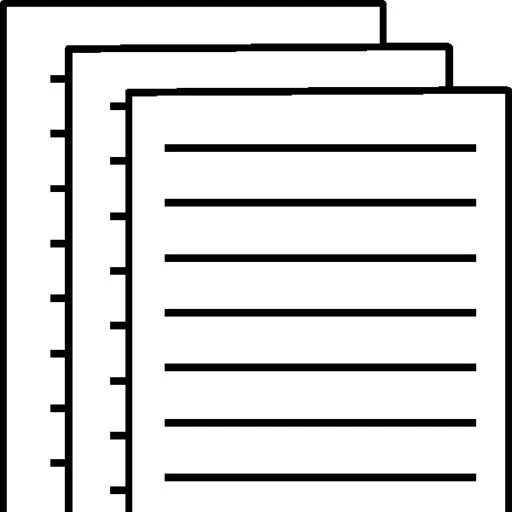## ACC100 AKEKS notes PIE 1 WEEK 4: Property plant and equipment section of a balance sheet Basic prob

Property, plant, and equipment section of a balance sheet: Basic problem

On January 2, 2017, Campbell Company purchased land that cost \$580,000, a buildingon the land that cost  \$1,320,000, and equipment that cost \$35,000. The building has an estimated useful life of 22 years. The equipment has an estimated useful life of 7 years.

Required:

Prepare the property, plant, and equipment section of the balance sheet as of December 31, 2017.

Note: Use straight-line depreciation with no salvage value

The property, plant, and equipment section of the balance sheet contains information about tangible assets that have useful lives longer than one year and are used in the operation of the business. Examples of these assets include land, buildingsequipment, furniture and fixtures, and other assets of a similar nature. These assets are reported at their book value.

In the property, plant, and equipment section of the balance sheet, all the fixed assets (except land) are reported at their historical cost (acquisition cost), less the related accumulated depreciation. Historical cost less accumulated depreciation is called book value. The book value of an asset should not be confused with its market value or replacement value. The book value is the value that the asset has on the accounting records (books).

Land:

Since land does not have a finite life, it is not depreciated. The amount reported on the balance sheet represents the land’s historical cost. Thus, the land is listed at \$580,000.

Buildings:

Since buildings have a finite life, they must be depreciated. To be depreciated means that the acquisition cost of the asset is allocated to the various periods in the asset’s life in a systematic and meaningful manner.

To calculate the amount depreciation, using the straight-line depreciation method, we use the following equation:

 Annual depreciation amount = (Cost – Salvage value)Useful life

As stated in the question, Campbell Company uses straight-line depreciation with no salvage value to determine the yearly depreciation. Since the salvage value is zero, to calculate the yearly depreciation, we take the cost of the building and divide it by the estimated number of years of useful life.

 Yearly depreciation = CostYears of life = \$1,320,00022 = \$60,000.

The balance in the contra-asset account, Accumulated Depreciation, Buildings, represents the first year’s depreciation. To calculate the book value of the building at the end of the first year, we take the cost of the building and subtract from it the balance in the Accumulated Depreciation, Buildings account.

 Book value of buildings = Buildings (cost) – Accumulated depreciation, buildings = \$1,320,000 – \$60,000 = \$1,260,000.

Equipment:

Since equipment has a finite life, it must be depreciated. To be depreciated means that the acquisition cost of the asset is allocated to the various periods in the asset’s life in a systematic and meaningful manner.

To calculate the amount of annual depreciation, using the straight-line depreciation method, we use the following equation:

 Annual depreciation amount = (Cost – Salvage value)Useful life

As stated in the question, Campbell Company uses straight-line depreciation with no salvage value to determine the yearly depreciation. Since the salvage value is zero, to calculate the yearly depreciation, we divide the cost of the equipment by the estimated number of years of useful life.

 Yearly depreciation = CostYears of life = \$35,0007 = \$5,000.

The balance in the contra-asset account, Accumulated Depreciation, Equipment, represents the first year’s depreciation. To calculate the book value of the equipment at the end of the first year, we take the cost of the equipment and subtract the balance in the Accumulated Depreciation, Equipment account.

 Book value of equipment = Equipment (cost) – Accumulated depreciation, equipment = \$35,000 – \$5,000 = \$30,000.

Now that we know the cost of the land and the book value of the buildings and the equipment, we can present the information. The total property, plant, and equipment section is equal to the cost of the land plus the book value of the buildings and the equipment.

 Total property, plant, and equipment = Land + Book value of buildings + Book value of equipment = \$580,000 + \$1,260,000 + \$30,000 = \$1,870,000

On January 2, 2017, Simmons Company purchased land that cost \$600,000, a buildingon the land that cost  \$1,410,000, and equipment that cost \$56,000. The building has an estimated useful life of 24 years. The equipment has an estimated useful life of 5 years.

Required:

Prepare the property, plant, and equipment section of the balance sheet as of December 31, 2017.

Note: Use straight-line depreciation with no salvage value.

On January 2, 2017, Kelly Company purchased land that cost \$640,000, a building on the land that cost \$1,030,000, and equipment that cost \$43,000. The building has an estimated useful life of 25 years. The equipment has an estimated useful life of 8 years.

Required:

Prepare the property, plant, and equipment section of the balance sheet as of December 31, 2017.

Note: Use straight-line depreciation with no salvage value

On January 2, 2017, Wright Company purchased land that cost \$830,000, a buildingon the land that cost  \$1,200,000, and equipment that cost \$28,000. The building has an estimated useful life of 20 years. The equipment has an estimated useful life of 10 years.

Required:

Prepare the property, plant, and equipment section of the balance sheet as of December 31, 2017.

Note: Use straight-line depreciation with no salvage value.

To view and download a complete answer, scroll down to the bottom to pay